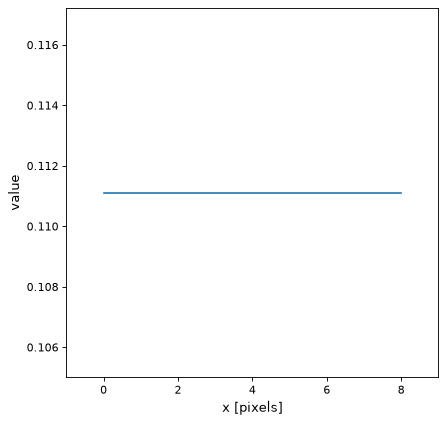# Box1DKernel¶

class `astropy.convolution.``Box1DKernel`(width, **kwargs)[source]

1D Box filter kernel.

The Box filter or running mean is a smoothing filter. It is not isotropic and can produce artifacts when applied repeatedly to the same data.

The generated kernel is normalized so that it integrates to 1.

By default the Box kernel uses the `linear_interp` discretization mode, which allows non-shifting, even-sized kernels. This is achieved by weighting the edge pixels with 1/2. E.g a Box kernel with an effective smoothing of 4 pixel would have the following array: [0.5, 1, 1, 1, 0.5].

Parameters
widthnumber

Width of the filter kernel.

mode`str`, optional
One of the following discretization modes:
• ‘center’

Discretize model by taking the value at the center of the bin.

• ‘linear_interp’ (default)

Discretize model by linearly interpolating between the values at the corners of the bin.

• ‘oversample’

Discretize model by taking the average on an oversampled grid.

• ‘integrate’

Discretize model by integrating the model over the bin.

factornumber, optional

Factor of oversampling. Default factor = 10.

Examples

Kernel response function:

```import matplotlib.pyplot as plt
from astropy.convolution import Box1DKernel
box_1D_kernel = Box1DKernel(9)
plt.plot(box_1D_kernel, drawstyle='steps')
plt.xlim(-1, 9)
plt.xlabel('x [pixels]')
plt.ylabel('value')
plt.show()
```

(png, svg, pdf)